### RESTRICT Statement

RESTRICT <’label’> constraint-specification <,, constraint-specification>
<operator <value>> </ option> ;

The RESTRICT statement enables you to specify linear equality or inequality constraints among the parameters of a mixture model. These restrictions are incorporated into the maximum likelihood analysis. The RESTRICT statement is not available for a Bayesian analysis with the FMM procedure.

Following are reasons why you might want to place constraints and restrictions on the model parameters:

• to fix a parameter at a particular value

• to equate parameters in different components in a mixture

• to impose order conditions on the parameters in a model

• to specify contrasts among the parameters that the fitted model should honor

A restriction is composed of a left-hand side and a right-hand side, separated by an operator. If the operator and right-hand side are not specified, the restriction is assumed to be an equality constraint against zero. If the right-hand side is not specified, the value is assumed to be zero.

An individual constraint-specification is written in (nearly) the same form as estimable linear functions are specified in the ESTIMATE statement of the GLM, MIXED, or GLIMMIX procedure. The constraint-specification takes the form

model-effect value-list < …model-effect value-list > <(SCALE= value)>

At least one model-effect must be specified followed by one or more values in the value-list. The values in the list correspond to the multipliers of the corresponding parameter that is associated with the position in the model effect. If you specify more values in the value-list than the model-effect occupies in the model design matrix, the extra coefficients are ignored.

To specify restrictions for effects in specific components in the model, separate the constraint-specification by commas. The following statements provide an example:

proc fmm;
class A;
model y/n = A x /  k = 2;
restrict A 1 0 -1;
restrict x 2, x -1 >= 0.5;
run;


The linear predictors for this two-component model can be written as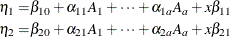where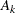is the binary variable associated with the kth level of A.

The first RESTRICT statement applies only to the first component and specifies that the parameter estimates that are associated with the first and third level of the A effect are identical. In terms of the linear predictor, the restriction can be written as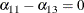Now suppose that A has only two levels. Then the FMM procedure ignores the value –1 in the first RESTRICT statement and imposes the restriction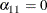on the fitted model.

The second RESTRICT statement involves parameters in two different components of the model. In terms of the linear predictors, the restriction can be written as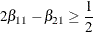When restrictions are specified explicitly through the RESTRICT statement or implied through the EQUATE=EFFECTS option in the MODEL statement, the FMM procedure lists all restrictions after the model fit in a table of linear constraints and indicates whether a particular constraint is active at the converged solution.

The following operators can be specified to separate the left- and right-hand sides of the restriction: =, >, <, >=, <=.

Some distributions involve scale parameters (the parameterin the expressions of the log likelihood) and you can also use the constraint-specification to involve a component’s scale parameter in a constraint. To this end, assign a value to the keyword SCALE, separated from the model effects and value lists with parentheses. The following statements fit a two-component normal model and restrict the component variances to be equal:

proc fmm;
model y = / k=2;
restrict int 0 (scale  1),
int 0 (scale -1);
run;


The intercept specification is necessary because each constraint-specification requires at least one model effect. The zero coefficient ensures that the intercepts are not involved in the restriction. Instead, the RESTRICT statement leads to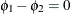.

You can specify the following option in the RESTRICT statement after a slash (/).

DIVISOR=value

specifies a value by which all coefficients on the right-hand side and left-hand side of the restriction are divided.